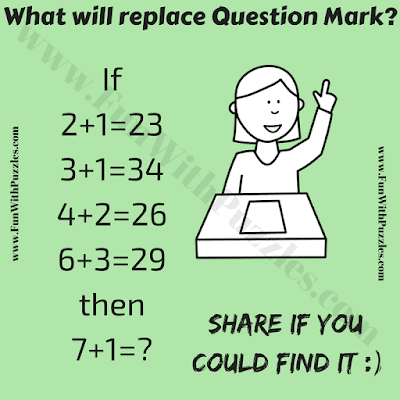## Sunday, December 8, 2019

This one is a tough brain teaser picture puzzle. In this brain teaser, there are some logical number equations are presented in the given puzzle picture. Each equation in the given puzzle picture following certain logical reasoning which makes each of these equations logical correct. Your challenge in this brain teaser picture puzzle is to decode the logical reasoning used in these equations and then solve the last given equation to find the missing number which will replace the question mark?Can you solve this Brain Teaser Picture Puzzle?

The answer to this "Brain Teaser Picture Puzzle", can be viewed by clicking on the button. Please do give your best try before looking at the answer.

1.1.Yes, you got answer to this brainteaser picture puzzle right.

2.what isis the logic

2.1.3.I got 38. 😅

1.2.count the number of non-vowel letters in the spellings for the first number.
two = 2
three = 3
four = 2
six = 2
SeVeN = 3, hence 3 + (7+1) = 38

4.It’s 38 because of a more complex and very consistent pattern. Add the 2 numbers on the left side of the equation, and put that number at the end... then is the 1st number on the left side is even, make the 1st number on the right side 2, and if it’s odd, make it 3.

applys to all of them

1.Great. Yes, your pattern applies to all the number in the given number equations. So your answer is also correct. Thanks for thinking outside the box and coming up with the different logical pattern.

5.6.7.8.9.